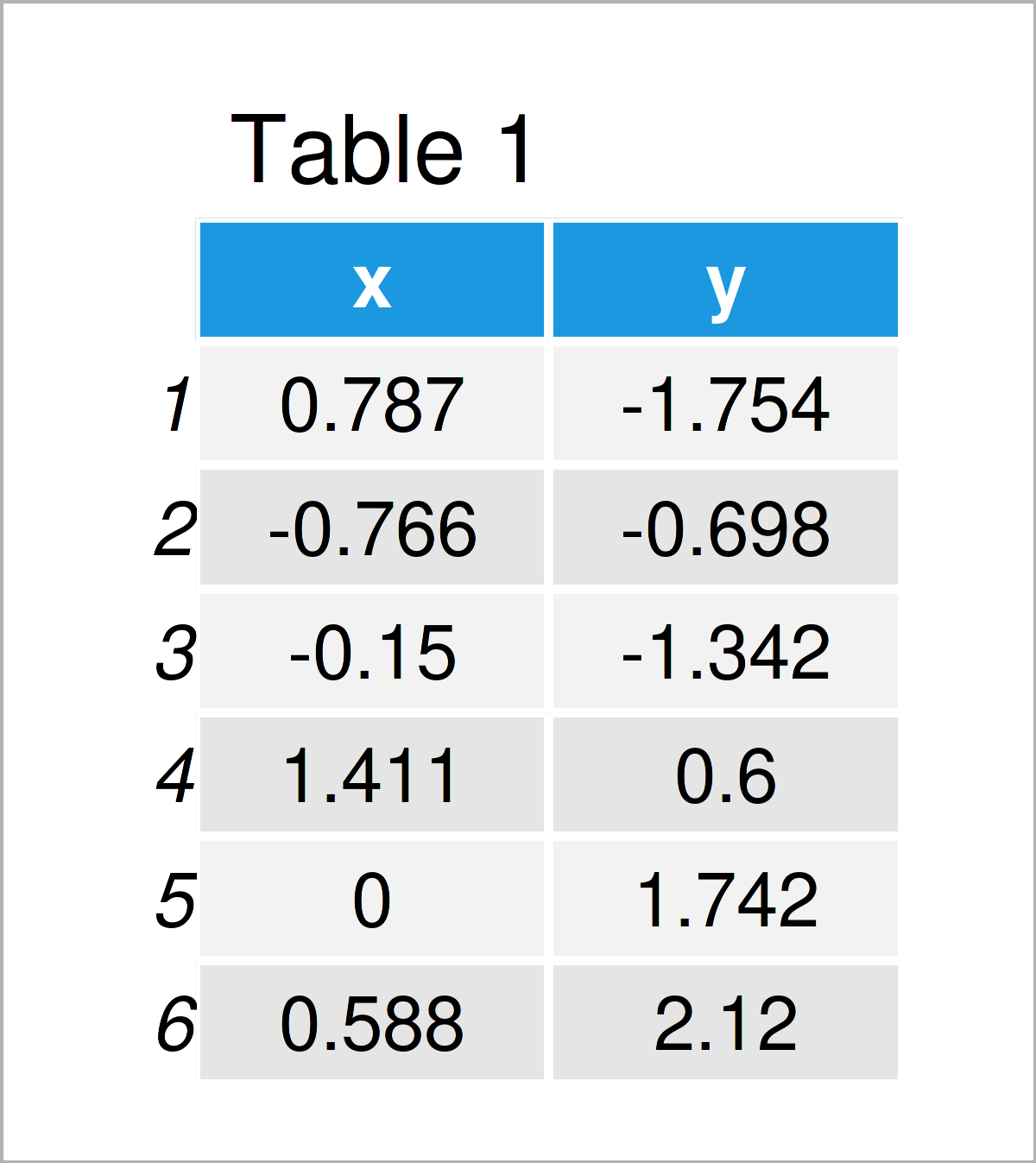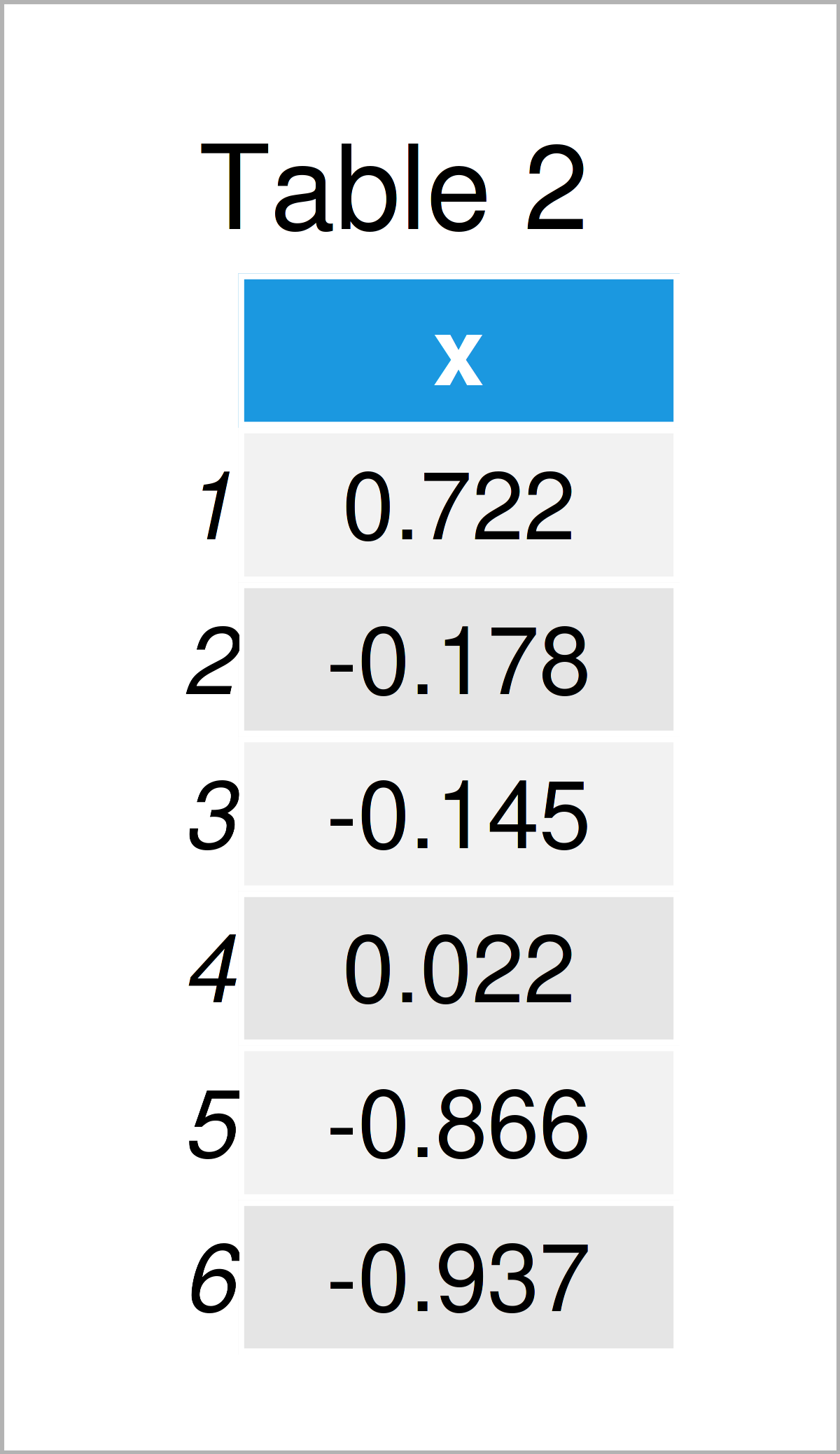# R Error in UseMethod(“predict”) : no applicable method for ‘predict’ applied to an object of class “c(‘double’, ‘numeric’)”

In this R tutorial you’ll learn how to handle the Error in UseMethod(“predict”) : no applicable method for ‘predict’ applied to an object of class “c(‘double’, ‘numeric’)”.

The page contains this:

Let’s just jump right in.

## Creating Example Data

First, let’s create some example data:

```set.seed(538946)                          # Create train data
data_train <- data.frame(x = rnorm(10),
y = rnorm(10))Table 1 visualizes the output of the RStudio console that got returned by the previous code and illustrates that our example data is composed of two numerical columns. This data frame will be used to train our model.

Next, we also have to create a test data frame:

```data_test <- data.frame(x = rnorm(10))    # Create test dataAs shown in Table 2, the previous R programming syntax has created another data frame that contains only the predictor column x.

## Example 1: Reproduce the Error in UseMethod(“predict”) : no applicable method for ‘predict’ applied to an object of class “c(‘double’, ‘numeric’)”

This section illustrates how to replicate the error message Error in UseMethod(“predict”) : no applicable method for ‘predict’ applied to an object of class “c(‘double’, ‘numeric’)”.

Let’s assume that we want to make predictions based on our data using the predict() function. Then, we might try to use the predict function as shown below:

```predict(data_test\$x, data_test)           # Try to predict values for test data
# Error in UseMethod("predict") :
#   no applicable method for 'predict' applied to an object of class "c('double', 'numeric')"```

Unfortunately, the previous R code has returned the Error in UseMethod(“predict”) : no applicable method for ‘predict’ applied to an object of class “c(‘double’, ‘numeric’)”.

This is because we have inserted a numeric column as first argument to the predict function instead of a model object.

Let’s solve this problem!

## Example 2: Fix the Error in UseMethod(“predict”) : no applicable method for ‘predict’ applied to an object of class “c(‘double’, ‘numeric’)”

Example 2 shows how to debug the Error in UseMethod(“predict”) : no applicable method for ‘predict’ applied to an object of class “c(‘double’, ‘numeric’)”.

For this, we first have to estimate a linear regression model based on our train data:

```mod <- lm(y ~ x, data_train)              # Estimate linear regression model
summary(mod)                              # Summary of linear regression model
# Call:
# lm(formula = y ~ x, data = data_train)
#
# Residuals:
#      Min       1Q   Median       3Q      Max
# -1.79523 -1.09487  0.05202  0.53017  2.10266
#
# Coefficients:
#             Estimate Std. Error t value Pr(>|t|)
# (Intercept) -0.05067    0.44588  -0.114    0.912
# x            0.11632    0.47348   0.246    0.812
#
# Residual standard error: 1.384 on 8 degrees of freedom
# Multiple R-squared:  0.007488,	Adjusted R-squared:  -0.1166
# F-statistic: 0.06035 on 1 and 8 DF,  p-value: 0.8121```

Next, we can apply the predict function to our model output and to our test data:

```predict(mod, data_test)                   # Properly to predict values for test data
#            1            2            3            4            5            6
#  0.033310908 -0.071341113 -0.067580482 -0.048135709 -0.151354152 -0.159618208
#            7            8            9           10
#  0.019528408 -0.160007711  0.003183706  0.035468402```

This time, the predict function worked perfectly.

Note that this error message may appear with small modifications. For instance, the error message Error in UseMethod(“predict”) : no applicable method for ‘predict’ applied to an object of class “train” occurrs when applying the predict function to a train object created by the caret package (see here), and the error message Error in UseMethod(“predict”) : no applicable method for ‘predict’ applied to an object of class “c(‘elnet’, ‘glmnet’)” is returned when applying the predict function to an elnet object (see here).

## Video & Further Resources

Have a look at the following video which I have published on my YouTube channel. In the video, I’m explaining the R syntax of this tutorial.

Furthermore, you might have a look at the other articles on my homepage. You can find a selection of tutorials below:

To summarize: At this point you should know how to reproduce and fix the Error in UseMethod(“predict”) : no applicable method for ‘predict’ applied to an object of class “c(‘double’, ‘numeric’)” in the R programming language. Please let me know in the comments section, in case you have any further questions and/or comments.

Subscribe to the Statistics Globe Newsletter

•Frank
May 25, 2023 1:11 pm

Hi Joachim, I was an early subscriber to your youtube channel and truly appreciate your content. I encountered a similar error I cannot resolve.

Glimpse of some variables in dataset:
\$ Bedrooms NA, 1, NA, 1, NA, NA, 1, NA, 1, NA, NA, 1, 4, NA, 2…
\$ Neighborhood.demo “Black”, “White”, “Latino”, “Unknown” ….
\$ Nightly.Rate , NA, 47.0, NA, 235.0, NA, 235.0, NA, NA, NA, NA, 104.5, NA, NA, N…

Model:
model.frac.logit4 <- glm(Vacancy.Rate ~ Bedrooms + Neighborhood.demo + Nightly.Rate, data = data, family = quasibinomial)

data_to_predict <- modelr::typical(model = model.frac.logit4,
Neighborhood.demo = c("Black", "Latino", "Unknown", "White"))

Error:
Error in UseMethod("typical") :
no applicable method for 'typical' applied to an object of class "c('glm', 'lm')"

•May 30, 2023 9:25 am

Hello Frank,

In the modelr package, the typical() function generates a “typical” dataset from a model, meaning it generates a new dataset with the same structure as the input to the model, but filled with typical values. This typical() function is not designed to handle glm or lm objects, but rather for vectors. The documentation is here. If you check the documentation, you may not find a specific implementation of the typical() function for these types of models.

Alternatively, you can create the “typical” data manually by creating a new data frame with the same structure as your original data. You can use the mean (or median or mode as appropriate) for numeric variables and the most common category for factor variables. Here is an example:

```getmode <- function(v) {
uniqv <- unique(v)
uniqv[which.max(tabulate(match(v, uniqv)))]
}

data_to_predict <- data.frame(
Bedrooms = mean(data\$Bedrooms, na.rm = TRUE),
Neighborhood.demo = getmode(data\$Neighborhood.demo),
Nightly.Rate = mean(data\$Nightly.Rate, na.rm = TRUE)
)```

This will give you a one-row data frame with the mean Bedrooms and Nightly.Rate and the mode Neighborhood.demo. I am unsure if it is the typical data that you want to use. But by definition, it is one way to do it. You can create your typical data manually based on your prediction purpose.

Please note that the mean and mode are computed using all data. NA values are ignored in the computation of the mean but are considered a value in the mode function and may be returned as the mode if they are the most common value. If you want to exclude NAs when computing the mode, you will need to modify the getmode() function or remove NA values before creating the typical data frame.

Regards,
Cansu